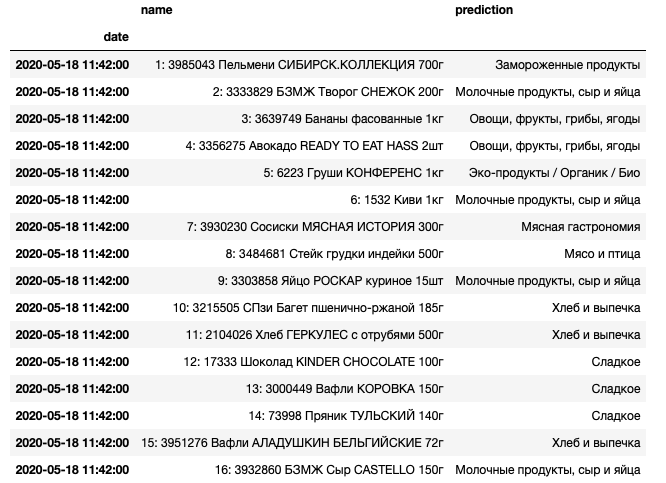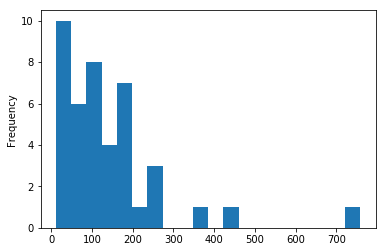3 posts tagged

# Predicting category of products by name from Russian Food Stores

Время чтения текста – 9 минут

This article is a continuation of our series about analyzing data on consumer products: «Collecting data from hypermarket receipts on Python» and «Parsing the data of site’s catalog, using Beautiful Soup and Selenium». We are going to build a model that would classify products by name in a till receipt. Till receipts contain data for each product bought, but it doesn’t provide us a summary of how much were spent on Sweets or Dairy Foods in total.

## Data Wrangling

Load data from our .csv file to a Pandas DataFrame and see how it looks:

Did you know that we can emulate human behavior to parse data from a web-catalog? More details about it are in this article: «Parsing the data of site’s catalog, using Beautiful Soup and Selenium»

``````import pandas as pdAs you can see, the DataFrame contains even more than we need for predicting the category of products by name. So we can drop() columns with prices and weights, and rename() the remaining ones:

``````sku.drop(columns=['Unnamed: 0', 'Weight','Price'],inplace=True)
sku.rename(columns={"SKU": "SKU", "Category": "Group"},inplace=True)Group the products by its category and count them up with the following methods:

``sku.groupby('Group').agg(['count'])``We will train our predictive model on this data so that it could identify the product category by name. Since the DataFrame includes product names mainly in Russian, the model won’t make predictions properly. The Russian language contains a lot of prepositions, conjunctions, and specific speech patterns. We want our model to distinguish that «Мангал с ребрами жесткости» («Brazier with strengthening ribs» ) and «Мангал с 6 шампурами» («Brazier with 6 skewers») belongs to the same category. With this is my we need to clean up all the product names, removing conjunctions, preposition, interjections, particles and keep only word bases with the help of stemming.

A stemmer is a tool that operates on the principle of recognizing “stem” words embedded in other words.

``````import nltk
from nltk.corpus import stopwords
from pymystem3 import Mystem
from string import punctuation

In our case will be using the pymystem3 library developed by Yandex. Product names in our DataFrame may vary from those ones you could find in supermarkets today. So first, let’s improve the list of stop words that our predictive model will ignore.

``````mystem = Mystem()
russian_stopwords = stopwords.words("russian")
russian_stopwords.extend(['лента','ассорт','разм','арт','что', 'это', 'так', 'вот', 'быть', 'как', 'в', '—', 'к', 'на'])``````

Write a function that would preprocess our data and extract the word base, remove punctuation, numerical signs, and stop words. The following code snippet belongs to one Kaggle kernel.

``````def preprocess_text(text):
text = str(text)
tokens = mystem.lemmatize(text.lower())
tokens = [token for token in tokens if token not in russian_stopwords\
and token != " " \
and len(token)>=3 \
and token.strip() not in punctuation \
and token.isdigit()==False]
text = " ".join(tokens)
return text``````

See how it works:

An extract from Borodino (Russian: Бородино), a poem by Russian poet Mikhail Lermontov which describes the Battle of Borodino.

``preprocess_text("Мой дядя самых честных правил, Когда не в шутку занемог, Он уважать себя заставил И лучше выдумать не мог.")``

Transformed into:

``'дядя самый честный правило шутка занемогать уважать заставлять выдумывать мочь'``

Everything works as expected – the result includes only word stems in lower case with no punctuation, prepositions or conjunctions. Let’s apply this function to a product name from our DataFrame:

``````print(‘Before:’, sku['SKU'])
print(‘After:’, preprocess_text(sku['SKU']))``````

Preprocessed text:

``````Before: Фисташки соленые жареные ТМ 365 дней
After: фисташка соленый жареный день``````

The function works fine and now we can apply it to the whole column, and create a new one with processed names:

``````sku['processed']=sku['SKU'].apply(preprocess_text)## Building our Predictive Model

We will be using CountVectorizer to predict the product category, and Naive Bayes Classifier.
CountVectorizer will tokenize our text and build a vocabulary of known words, while Naive Bayes Classifier allows us to train our model on a DataFrame with multiple classes. We will also need TfidfTransformer for computing words count (term frequency). As we want to chain these steps, let’s import the Pipeline library:

``````import pandas as pd
from sklearn.model_selection import train_test_split
from sklearn.feature_extraction.text import CountVectorizer
from sklearn.feature_extraction.text import TfidfTransformer
from sklearn.naive_bayes import MultinomialNB
from imblearn.pipeline import Pipeline``````

Separate our targets, Y (categories) from the predictors, X (processed product names). And split the DataFrame into Test and Training sets, allocating 33% of samples for testing.

``````x = sku.processed
y = sku.Group
X_train, X_test, y_train, y_test = train_test_split(x, y, test_size=0.33)``````

Add the following methods to our pipeline:

• CountVectorizer() – returns a matrix of token counts
• TfidfTransformer() – transforms a matrix into a normalized tf-idf representation
• MultinomialNB() – an algorithm for predicting product category
``````text_clf = Pipeline([('vect', CountVectorizer(ngram_range=(1,2))),
('tfidf', TfidfTransformer()),
('clf', MultinomialNB())])``````

Fit our model to the Training Dataset and make predictions for the Test Dataset:

``````text_clf = text_clf.fit(X_train, y_train)
y_pred = text_clf.predict(X_test)``````

Evaluate our predictive model:

``print('Score:', text_clf.score(X_test, y_test))``

The model predicts correctly 90% of the time.

``Score: 0.923949864498645``

## Validate our model with the real-world data

Let’s test how good our model performs on real-world data. We’ll refer to the DataFrame from our previous article, «Collecting data from hypermarket receipts on Python», and preprocess the product names:

``````my_products['processed']=my_products['name'].apply(preprocess_text)Pass the processed text to the model and create a new column that would hold our predictions:

``````prediction = text_clf.predict(my_products['processed'])
my_products['prediction']=prediction
my_products[['name', 'prediction']]``````

Now, the DataFrame looks the following:Calculate the spendings for each product category:

``my_products.groupby('prediction').sum()``Overall, the model seems to be robust in predicting that sausages fall under meat products, quark is a dairy product, baguette belongs to bread and pastries. But sometimes it misclassifies kiwi as a dairy product and pear as an eco-product. This is probably because these categories include many products are «with the taste of pear» or «with the taste of kiwi», and the algorithm makes predictions based on the prevailing group of products. This is a well-known issue of unbalanced classes, but it can be addressed by resampling the DataSet or choosing proper weights for our model.

No comments    260   2020   data analytics   machine learning   python

# Map of Machine Learning algorythms

Время чтения текста – 1 минута

Couple of days ago I’ve seen that scikit-learn posted a nice map of machine learning algorythms.No comments    101   2019   machine learning   python

# Collecting data from hypermarket receipts on Python

Время чтения текста – 6 минут

Recently, once again buying products in a hypermarket, I recalled that, according to the Russian Federal Act FZ-54, any trade operator, that issues a receipt, is obliged to send the data thereof to the Tax Service.Receipt from “Lenta” hypermarket. The QR-code of our interest is circled.

So, what does it mean for us, data analysts? It means that we can know ourselves and our needs better, and also acquire interesting data on own purchases.

Let’s try to assemble a small prototype of an app that will allow to make a dynamic of our purchases within the framework of blog posts’ series. So, we’ll start from the fact, that each receipt has a QR-code, and if you identify it, you’ll receive the following line:

t=20190320T2303&s=5803.00&fn=9251440300007971&i=141637&fp=4087570038&n=1

This line comprises:

t – timestamp, the time when you made a purchase
s – sum of the receipt
fn – code number of fss, will be needed further in a request to API
i – receipt number, will be needed further in a request to API
fp – fiscalsign parameter, will be needed further in a request to API

Within the solution of the first step, we will parse the receipt data and collect it in pandas dataframe, using Python modules.

We will use API, that provides data on the receipt from the Tax Service website.

Initially, we will receive authentication data:

``````import requests
your_phone = '+7XXXYYYZZZZ' #you need to state your phone number, SMS with password will arrive thereon
r = requests.post('https://proverkacheka.nalog.ru:9999/v1/mobile/users/signup', json = {"email":"email@email.com","name":"USERNAME","phone":your_phone})``````

As a result of performing POST request we receive a password in SMS to the indicated phone number. Further on, we will be using it in a variable pwd

Now we’ll parse our line with values from QR-code:

``````import re
qr_string='t=20190320T2303&s=5803.00&fn=9251440300007971&i=141637&fp=4087570038&n=1'
t=re.findall(r't=(\w+)', qr_string)
s=re.findall(r's=(\w+)', qr_string)
fn=re.findall(r'fn=(\w+)', qr_string)
i=re.findall(r'i=(\w+)', qr_string)
fp=re.findall(r'fp=(\w+)', qr_string)``````

We’ll use the variables obtained in order to extract the data.
One Habr post pretty thoroughly examines status of errors at formation of API request, therefore I won’t repeat this information.

In the beginning, we need to verify the presence of data on this receipt, so we form a GET request.

``````headers = {'Device-Id':'', 'Device-OS':''}
payload = {'fiscalSign': fp, 'date': t,'sum':s}
print(check_request.status_code)``````

In the request one needs to indicate headers, at least empty ones. In my case, GET request returns error 406, thus I get that such receipt is found (why GET request returns 406 remains a mystery to me, so I will be glad to receive some clues in comments). If not indicating sum or date, GET request returns error 400 – bad request.

Let’s move on to the most interesting part, receiving data of the receipt:

``````request_info=requests.get('https://proverkacheka.nalog.ru:9999/v1/inns/*/kkts/*/fss/'+fn+'/tickets/'+i+'?fiscalSign='+fp+'&sendToEmail=no',headers=headers,auth=(your_phone, pwd))
print(request_info.status_code)
products=request_info.json()``````

We should receive code 200 (successful execution of the request), and in the variable products – everything, that applies to our receipt.

In order to further work with this data, let’s use pandas and transform everything in dataframe.

``````import pandas as pd
from datetime import datetime
my_products=pd.DataFrame(products['document']['receipt']['items'])
my_products['price']=my_products['price']/100
my_products['sum']=my_products['sum']/100
datetime_check = datetime.strptime(t, '%Y%m%dT%H%M') #((https://docs.python.org/3/library/datetime.html#strftime-and-strptime-behavior formate the date))
my_products['date']=datetime_check
my_products.set_index('date',inplace=True)``````

Now we have working pandas.dataframe with receipts, visually it looks as follows:You can construct a bar chart of purchases or observe everything as a box plot:

``````import matplotlib.pyplot as plt
%matplotlib inline
my_products['sum'].plot(kind='hist', bins=20)
plt.show()
my_products['sum'].plot(kind='box')
plt.show()``````boxplot_cheques.png

In conclusion, we will simply get descriptive statistics as text, using a command .describe():

``my_products.describe()``It’s convenient to write down data as .csv file, so that the next time you can amend the statistics:

``````with open('hyper_receipts.csv', 'a') as f: